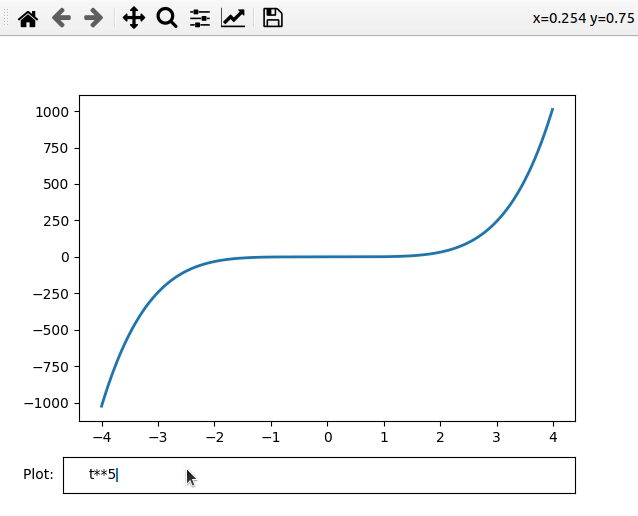Related Articles
Matplotlib – Textbox Widgets
• Last Updated : 24 Feb, 2021

In this article, we are going to see matplotlib’s textbox widget. Matplotlib is a plotting library for the Python programming language. In this article, we will try to plot a graph for different powers(e.g. t^2, t^3, t^9, etc.) using textbox widget.

The Textbox is a widget that accepts input from the user. Input can also be formulas so that we can generate a graph based on those formulas. To use this widget we use TextBox() function.

It accepts two parameters

• Figure i.e. graph to use.
• Text to display with textbox i.e. Label to txtBox.

Below is the implementation of this widget:

## Python

 `# Import modules``import` `numpy as np``import` `matplotlib.pyplot as plt``from` `matplotlib.widgets ``import` `TextBox`` ` `# Adjust illustration``fig, ax ``=` `plt.subplots()``fig.subplots_adjust(bottom``=``0.2``)`` ` `t ``=` `np.arange(``-``4.0``, ``4.0``, ``0.01``)``l, ``=` `ax.plot(t, np.zeros_like(t), lw``=``2``)`` ` `# Function to plot graph ``# according to expression``def` `visualizeGraph(expr):``    ``ydata ``=` `eval``(expr)``    ``l.set_ydata(ydata)``    ``ax.relim()``    ``ax.autoscale_view()``    ``plt.draw()`` ` `# Adding TextBox to graph``graphBox ``=` `fig.add_axes([``0.1``, ``0.05``, ``0.8``, ``0.075``])``txtBox ``=` `TextBox(graphBox, ``"Plot: "``)``txtBox.on_submit(visualizeGraph)``txtBox.set_val(``"t**5"``)`` ` `# Plot graph``plt.show()`

Output:Explanation:

In the above program, we created a graph for t2 as by default graph. In txtBox labelled as Plot: we can enter the formula we want to view the graph for.

Attention geek! Strengthen your foundations with the Python Programming Foundation Course and learn the basics.

To begin with, your interview preparations Enhance your Data Structures concepts with the Python DS Course.

My Personal Notes arrow_drop_up